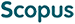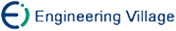杨 剑, 王 珏, 钟 宁. 流形上的Laplacian半监督回归[J]. 计算机研究与发展, 2007, 44(7): 1121-1127.
 引用本文: 杨 剑, 王 珏, 钟 宁. 流形上的Laplacian半监督回归[J]. 计算机研究与发展, 2007, 44(7): 1121-1127.Yang Jian, Wang Jue, Zhong Ning. Laplacian Semi-Supervised Regression on a Manifold[J]. Journal of Computer Research and Development, 2007, 44(7): 1121-1127.
 Citation: Yang Jian, Wang Jue, Zhong Ning. Laplacian Semi-Supervised Regression on a Manifold[J]. Journal of Computer Research and Development, 2007, 44(7): 1121-1127.## Laplacian Semi-Supervised Regression on a Manifold

• 摘要: 把流形学习与半监督学习相结合，研究了流形上的半监督回归问题.简要介绍了半监督流形学习的Laplacian正则化框架，在此基础上推导了基于一类广义损失函数的Laplacian半监督回归，它能够利用数据所在流形的内在几何结构进行回归估计.具体给出了线性ε-不敏感损失函数，二次ε-不敏感损失函数和Huber损失函数的Laplacian半监督回归算法，在模拟数据和Boston Housing数据上对算法进行了实验，并对实验结果进行了分析.这些结果将为进一步深入研究半监督流形回归问题提供一些可借鉴的积累.

Abstract: Recently, manifold learning and semi-supervised learning are two hot topics in the field of machine learning. But there are only a few researches on semi-supervised learning from the point of manifold learning, especially for semi-supervised regression. In this paper, semi-supervised regression on manifolds is studied, which can employ the manifold structure hidden in datasets to the problem of regression estimation. Firstly the framework of Laplacian regularization presented by M. Belkin et al. is introduced. Then the framework of Laplacian semi-supervised regression with a class of generalized loss functions is deduced. Under this framework, Laplacian semi-supervised regression algorithms with linear ε-insensitive loss functions, quadric ε-insensitive loss functions and Huber loss functions are presented. Their experimental results on S-curve dataset and Boston Housing dataset are given and analyzed. The problem of semi-supervised regression on manifolds is interesting but quite difficult. The aim of this paper is only to accumulate some experience for further research in the future. There are still many hard problems on semi-supervised regression estimation on manifolds, such as constructing statistical basis of the algorithm, looking for better graph regularizer in the framework of Laplacian semi-supervised regression, designing quicker algorithms, implementing the algorithm on more datasets and so on./下载:  全尺寸图片 幻灯片
• 分享
• 用微信扫码二维码

分享至好友和朋友圈